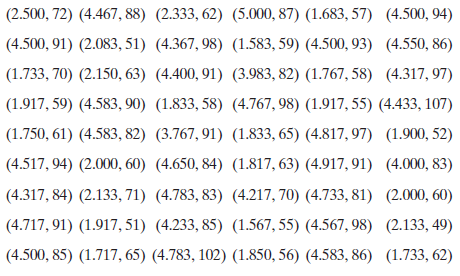×
Get Full Access to Probability And Statistical Inference - 9 Edition - Chapter 7.7 - Problem 6e
Get Full Access to Probability And Statistical Inference - 9 Edition - Chapter 7.7 - Problem 6e

×

# The following 54 pairs of data give, for Old FaithfulISBN: 9780321923271 41

## Solution for problem 6E Chapter 7.7

Probability and Statistical Inference | 9th Edition

• Textbook Solutions
• 2901 Step-by-step solutions solved by professors and subject experts
• Get 24/7 help from StudySoup virtual teaching assistantsProbability and Statistical Inference | 9th Edition

4 5 1 274 Reviews
25
1
Problem 6E

The following 54 pairs of data give, for Old Faithful geyser, the duration in minutes of an eruption and the time in minutes until the next eruption:(a) Calculate the correlation coefficient, and construct a scatterplot, of these data.(b) To estimate the distribution of the correlation coefficient,, resample 500 samples of size 54 from the empirical distribution, and for each sample, calculate the value of.(c) Construct a histogram of these 500 observations of.(d) Simulate 500 samples of size 54 from a bivariate normal distribution with correlation coefficient equal to the correlation coefficient of the geyser data. For each sample of 54, calculate the correlation coefficient.(e) Construct a histogram of the 500 observations of the correlation coefficient.(f) Construct aplot of the 500 observations offrom the bivariate normal distribution of part (d) versus the 500 observations in part (b). Do the two distributions ofappear to be about equal?

Step-by-Step Solution:
Step 1 of 3

Chapter 8: bivariate correlational research - When you see two things that are related, and you think are related, but actually have nothing to do with one another To be causal - Covariance - Internal validity - Temporal precedence Correlational strength - As you get a measurement change by this much on this chart or whatever - You get the same ratio change on the other chart for the other variable - This means that they are changing at the same rate o Either moving at the same amount in the same direction [perfect correlation o Or moving at the same amount in the opposite direction [perfect correlation - When they don’t move at the same rate; imperfect correlation – this is what r

Step 2 of 3

Step 3 of 3

##### ISBN: 9780321923271

Unlock Textbook Solution